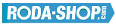# Remote ControlManufacturer+ Product Image Item Name Price### 30/5-2RS

Miniature Metric Series Chrome Steel Rubber Seals on Both sides Dimension d=5.00 Dimension D=14.00 Dimension B=7.00 Dinamic Load Rating=2020 Static Load Rating=930 Weight=0.008gr. Speed limit Grease=30.0 Speed limit Oil=38.0
inside diameter = 5.00
outside diameter = 14.00
width = 7.00
€9.62### 30/5-2Z

Miniature Metric Series Chrome Steel Metallic Shields on both sides Dimension d=5.00 Dimension D=14.00 Dimension B=7.00 Dinamic Load Rating=2020 Static Load Rating=930 Weight=0.008gr. Speed limit Grease=30.0 Speed limit Oil=38.0
inside diameter = 5.00
outside diameter = 14.00
width = 7.00### 30/6-2RS

Miniature Metric Series Chrome Steel Rubber Seals on Both sides Dimension d=6.00 Dimension D=17.00 Dimension B=6.00 Dinamic Load Rating=3080 Static Load Rating=1450 Weight=0.01gr. Speed limit Grease=30.0 Speed limit Oil=39.0
inside diameter = 6.00
outside diameter = 17.00
width = 6.00
€22.51### 30/7-2RS

Miniature Metric Series Chrome Steel Rubber Seals on Both sides Dimension d=7.00 Dimension D=19.00 Dimension B=10.00 Dinamic Load Rating=3650 Static Load Rating=2000 Weight=0.012gr. Speed limit Grease=28.0 Speed limit Oil=30.0
inside diameter = 7.00
outside diameter = 19.00
width = 10.00### 30/7-2Z

Miniature Metric Series Chrome Steel Metallic Shields on both sides Dimension d=7.00 Dimension D=19.00 Dimension B=10.00 Dinamic Load Rating=3650 Static Load Rating=2000 Weight=0.012gr. Speed limit Grease=28.0 Speed limit Oil=30.0
inside diameter = 7.00
outside diameter = 19.00
width = 10.00### 30/8-2RS

Miniature Metric Series Chrome Steel Rubber Seals on Both sides Dimension d=8.00 Dimension D=22.00 Dimension B=11.00 Dinamic Load Rating=5070 Static Load Rating=2580 Weight=0.02gr. Speed limit Grease=30.0 Speed limit Oil=36.0
inside diameter = 8.00
outside diameter = 22.00
width = 11.00
€12.51### 30/8-2Z

Miniature Metric Series Chrome Steel Metallic Shields on both sides Dimension d=8.00 Dimension D=22.00 Dimension B=11.00 Dinamic Load Rating=5070 Static Load Rating=2580 Weight=0.02gr. Speed limit Grease=30.0 Speed limit Oil=36.0
inside diameter = 8.00
outside diameter = 22.00
width = 11.00### 601X

Miniature Metric Series Chrome Steel Open(No Shields) Dimension d=1.50 Dimension D=6.00 Dimension B=2.50 Dinamic Load Rating=330 Static Load Rating=99 Weight=0.001gr. Speed limit Grease=75.0 Speed limit Oil=90.0
inside diameter = 1.50
outside diameter = 6.00
width = 2.50
€2.24### 601X-2Z

Miniature Metric Series Chrome Steel Metallic Shields on both sides Dimension d=1.50 Dimension D=6.00 Dimension B=3.00 Dinamic Load Rating=330 Static Load Rating=99 Weight=0.001gr. Speed limit Grease=75.0 Speed limit Oil=90.0
inside diameter = 1.50
outside diameter = 6.00
width = 3.00
€2.24### 602X

Miniature Metric Series Chrome Steel Open(No Shields) Dimension d=2.50 Dimension D=8.00 Dimension B=2.80 Dinamic Load Rating=552 Static Load Rating=177 Weight=0.001gr. Speed limit Grease=60.0 Speed limit Oil=71.0
inside diameter = 2.50
outside diameter = 8.00
width = 2.80
€2.24